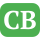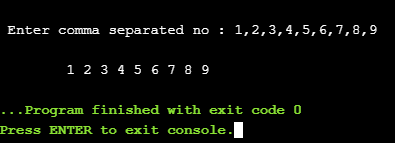CppBuzz.com

# C++ program to split vector into groups

This C++ program takes comman separated string as input, parse it into Vector of int and then print it in groups of 2 and 1 values.

``````#include
#include <string>
#include <sstream>
#include <iostream>

void print2values(int a, int b);
void print1value(int a);

int main()
{
std::string str;
std::cout<<"\n Enter comma separated no : ";
std::cin>>str;

std::vector vect;
std::stringstream ss(str);

int i;

std::cout<<"\n\t";

while (ss >> i)
{
vect.push_back(i);

if (ss.peek() == ',')
ss.ignore();
}

for (i=0; i< vect.size(); i++)

if(i <= vect.size()-2){
print2values(vect.at(i), vect.at(i+1));
i++;
}
else{
print1value(vect.at(i));
}

}

void print2values(int a, int b){
std::cout<<a<<" "<<b<<" ";
}

void print1value(int a){
std::cout<<a<<" ";
}```
```

## Grouping of vector elements